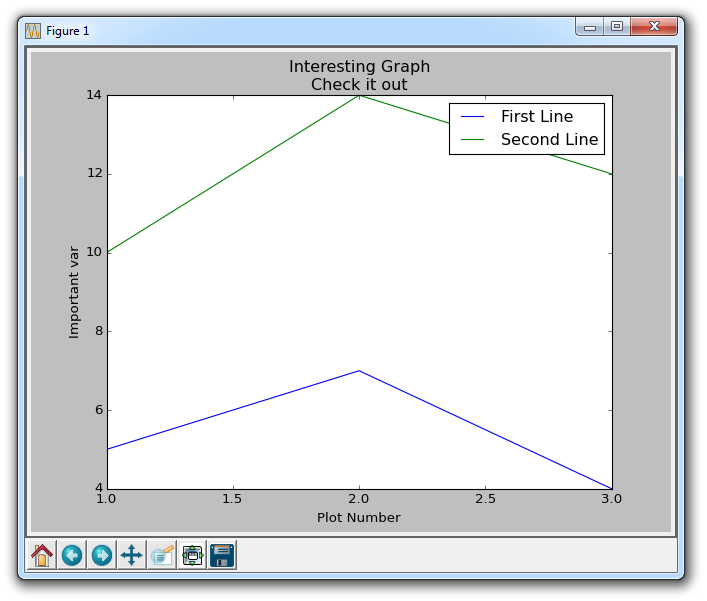# 第二章 图例、标题和标签

``````import matplotlib.pyplot as plt

x = [1,2,3]
y = [5,7,4]

x2 = [1,2,3]
y2 = [10,14,12]
``````

``````plt.plot(x, y, label='First Line')
plt.plot(x2, y2, label='Second Line')
``````

``````plt.xlabel('Plot Number')
plt.ylabel('Important var')
plt.title('Interesting Graph\nCheck it out')
plt.legend()
plt.show()
``````